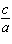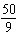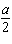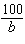Name:    Quiz 1.1-1.3

Multiple Choice
Identify the choice that best completes the statement or answers the question.

Write an expression for the problem.

1.

You buy 3 kick sacks at \$2 each. How much money do you spend?
 a. Total cost = 3 ÷ 2 c. Total cost = 3 • 2 b. Total cost = 3 • 2 • 3 d. Total cost = 2 ÷ 3

2.

 a. Trading cards now = 88 ÷ 14 c. Trading cards now = 88 + 14 b. Trading cards now = 88 – 14 d. Trading cards now = 88 • 14

Evaluate the expression when a = 5, b = 9, and c = 10.

3.

1 + a
 a. 6 c. 4 b. 8 d. 5

4.

3a
 a. 18 c. 20 b. 15 d. 12

Evaluate the expression when a = 9, b = 4, and c = 36.

5.

c a
 a. 29 c. 32 b. 28 d. 27

6.a. 3 c.b. 4 d. 5

Evaluate the expression when a = 10, b = 9, and c = 50.

7.

a + b
 a. 60 c. 59 b. 19 d. 17

8.a.c. 5 b. 3 d. 6

9.

a • b
 a. 90 c. 88 b. 92 d. 60

Tell which property is illustrated by the statement.

10.

c • 1 = c
 a. Multiplication Property of One c. Commutative Property of Multiplication b. Distributive Property d. Associative Property of Multiplication

Simplify the expression.

11.

20 + (12 + x)
 a. 12 + x c. 32x b. 32 + x d. 20 + x

12.

6 + (0 + p)
 a. 6 c. 6 + p b. 6p d. 0

13.

The expression 16 + x + 22 represents the perimeter of a triangle. Simplify the expression.
 a. 38 c. 54 b. x + 38 d. 38x

Numeric Response

Evaluate the expression when a = 14, b = 10, and c = 8.

1.+ 12

2.+ 8